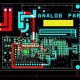# What is Sequential Circuit & Its Types?

In this tutorial, we are going to learn about Sequential Circuit & Its Types.

Sequential Circuit –

• It is a logical circuit, which is the output depends on the present value of the input as well as the sequence of past inputs/outputs.
• Sequential Circuit is a combinational circuit with memory.
• The output of Sequential Circuit depends upon present inputs and present state.
• As per below diagram of Sequential Circuit can see the information stored in sequential circuit represents present state.
• The present state and present inputs will define output and input state.

Below is the main point of Sequential Circuit –

• Output Variable at any instant of time depends not only on present input but also on previous outputs.
• Memory Unit is required for this circuit.
• It is slow circuit.
• It is design to difficult.
• In this circuit, The present output depends on the present input as well as past output/outputs.

Types of Sequential Circuit –

1. Asynchronous –
2. Synchronous –
• Below are the table for Synchronous :

Below are the table for Asynchronous :